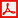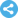International Journal of Mathematical Analysis and Applications
Manuscript Information

Improving Analytic Function Approximation by Minimizing Square Error of Taylor Polynomial
International Journal of Mathematical Analysis and Applications
Vol.1 , No. 4, Publication Date: Oct. 21, 2014, Page: 63-67
1464 Views Since October 21, 2014, 440 Downloads Since Apr. 14, 2015Paper in PDF (134K)Follow on

Authors

  Loc Nguyen, Vietnam Institute of Mathematics, Hanoi, Vietnam.

Abstract

It is very necessary to represent arbitrary function as a polynomial in many situations because polynomial has many valuable properties. Fortunately, any analytic function can be approximated by Taylor polynomial. The quality of Taylor approximation within given interval is dependent on degree of Taylor polynomial and the width of such interval. Taylor polynomial gains highly precise approximation at the point where the polynomial is expanded and so, the farer from such point it is, the worse the approximation is. Given two successive Taylor polynomials which are approximations of the same analytic function in given interval, this research proposes a method to improve the later one by minimizing their deviation so-called square error. Based on such method, the research also propose a so-called shifting algorithm which results out optimal approximated Taylor polynomial in given interval by dividing such interval into sub-intervals and shifting along with sequence of these sub-intervals in order to improve Taylor polynomials in successive process, based on minimizing square error.

Keywords

Taylor Polynomial, Analytic Function Approximation, Square Error

Reference

  [Burdden 2011]. Burdden, R. L., Faires, J. D. Numerical Analysis, ninth edition. Brooks/Cole Cengage Learning Publisher Copyright 2011, 2005, 2001.  [Callahan 2008] James Callahan, J., Hoffman, K., Cox, D., O’Shea, D., Pollatsek, H., Senechal, L. Calculus in Context. The Five College Calculus Project Copyright 1994, 2008.  [Jia 2013]. Jia, Y. B. Lagrange Multipliers. Lecture notes on course “Problem Solving Techniques for Applied Computer Science”, Iowa State University of Science and Technology, USA. Last checked 2 September 2014.  [Montgomery 2003]. Montgomery, D. C., Runger, G. C. Applied Statistics and Probability for Engineers, third edition. John Wiley & Sons Publisher Copyright 2003.  [Nguyen 1999]. Nguyen, H. V. H. Linear Algebra, second edition. Hanoi Education Publisher Copyright 1999.